# Survival Analysis: Comparison of Two Survival Curves Allowing for Stratification

Hypothesis: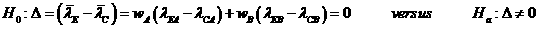Data Input: (Help) (Example)

 Input Results Stratum A Stratum B Stratum A Stratum B α N β DC K DE δ DT MS QC NT QE NC T0 NE T-T0

Note:

 Variables Descriptions α Significance level 1-β Power of the test K Proportion of sample in each stratum δ Minimum hazards ratio,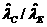, the ratio of two estimated hazard rates MS Control median survival (month) QC Proportion in control group QE Proportion in experimental group T0 Accrual duration (month) T Length of study (month) T-T0 Follow-up duration (month) N Sample size in each stratum DC Number of deaths in control group DE Number of deaths in experimental group DT Total number of deaths in each stratum NT Total sample size for all strata NC Sample size for control group NE Sample size for experimental group

Help Aids

Application:  The determination of the number of patients needed in a prospective comparison of survival curves in stratified analysis, when the control group patients have already been followed for some period, following hypotheses with two strata are usually used:where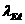is the hazard rate of patients in experimental group in stratum A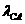is the hazard rate of patients in control group in stratum A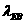is the hazard rate of patients in experimental group in stratum B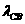is the hazard rate of patients in control group in stratum B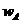is the weight of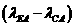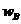is the weight of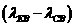Procedure:

1. Enter

a)   value of α, the probability of type I error

b)   value of β, the probability of type II error, or (1-power) of the test

c)   value of K, the proportion of sample in each stratum, the sum of K’s in all strata must be equal to 1

d)   value of δ, the minimum hazards ratio, it is specified in alternate hypothesis

e)   value of MS, the control median survival (month), it is estimated from existing control data

f)   value of QC, the proportion of patient in control group compared with experimental group in each stratum

g)   value of QE, the proportion of patient in experimental group compared with control group in each stratum, where QC + QE = 1

h)   value of T0, accrual duration (month), the length of time to recruit patients for study in each stratum

i)    value of T-T0, follow-up duration (month, the length of study time of all recruited patients to the end of study T in each stratum

1. Click the button “Calculate” to obtain

a)    value of N, the sample size of patients in the experimental arm in each stratum

b)    value of DC, the number of deaths in control group in each stratum

c)    value of DE, the number of deaths in experimental group in each stratum

d)     value of DT, the total number of deaths at the end of study in each stratum

e)    value of NT, the total sample size for all strata

f)    value of NC, the total sample size for control group for all strata

g)    value of NE, the total sample size for experimental group for all strata

Assumption:

1.      Time to survival is exponential distributed with hazard rate λ.

Theory:

In some cases, the trial may be designed with the intent of conducting a pooled (e.g., Mantel-Haenszel) analysis over strata. For simplicity we consider the case of two strata, say A and B, with sample sizes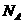and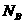(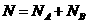), where each stratum may have an associated recruitment and follow-up period (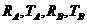) and associated hazards under the alternative hypothesis (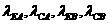), For example, strata A and B may correspond to a prognostic factor (e.g., severity of disease), or stratum A  mat refer to a feasibility or pilot phase and stratum B to the main trial.

For each cases, the test statistic would employ pooled estimators of the within-stratum differences in hazard rated: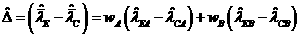, where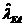,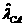are the estimated hazards from stratum A ofpatients, and likewise for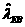,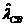ofpatients. Further, let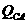,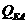be the sample fractions of the two treated groups in stratum A (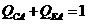), and likewise,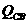,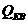for stratum B.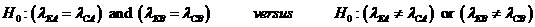Under the assumption of uniform entry with no losses to follow-up,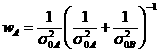,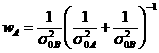where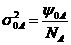,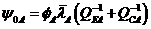,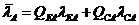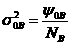,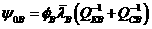,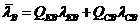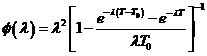Under the null hypothesis, it then follows that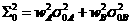Under the alternative hypothesis,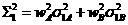where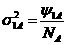,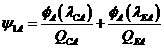and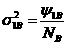,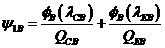If we then express the strata samples sizes as fractions of the total,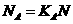,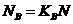, it is easily shown that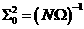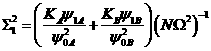where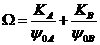The basic equation relating sample size and power iswhere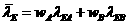,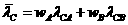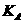and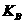are the strata sample fractions (e.g.,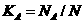)

Notations:

α:         The probability of type I error (significance level) is the probability of rejecting the true null hypothesis.

β:         The probability of type II error (1 – power of the test) is the probability of not rejecting the false null hypothesis.

Example:

Consider the case of two prognostic strata, such as early-versus late-stage disease, with stratum-specific hazards and relative strata size KA=0.43 and KB=0.57. Suppose a clinical trial is to be conducted for a disease with moderate levels of mortality with hazard rare 0.035 in all strata (λCA= λCB=0.035), yielding 50% survivors after 20 months (MSA= MSB=20). Suppose that with treatment we are interested in detecting a half reduction in hazard rate in all strata (δA=δB=2).

It is a two sided test with equal-sized group (QCA=QEA=QCB=QEB=0.5), significance level 5% (α =0.05) with power 80% power (β=0.2), and assume that recruitment was to be terminated after 56 months of a 124-month study in stratum A (TA=124, T0A=56, TA-T0A=68) and 56 months of a 68-month study in stratum B (TB=68, T0B=56, TB-T0B=12), then the required sample size is 42 and 56 for stratum A and B (NA=42, NB=55). The total number of deaths is 37 and 33 for stratum A and B (DTA=42, DTB=55). The total number for control group is 49 (NC=49) and for experimental group is 49 (NE=49).

Reference:

1. Lachin and Foulkes (1986) Biometrics 42: 507-519